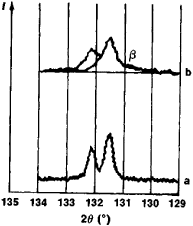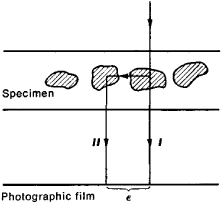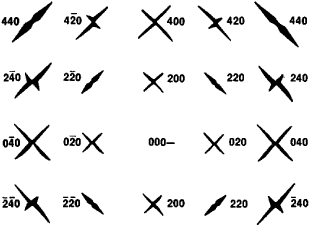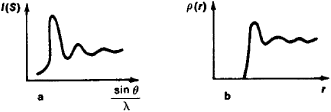# X-Ray Diffraction Analysis of Materials

## X-Ray Diffraction Analysis of Materials

the field of research dealing with the solution of diverse problems in materials science on the basis of X-ray diffraction techniques. Materials in both equilibrium and nonequilibrium states are investigated with respect to their crystalline structure, phase composition, and variations thereof; phase diagrams are constructed, and the state of deformed materials or of materials subjected to other actions, ordering processes, and phenomena of short-range order in materials are also investigated.

X-ray analysis of materials uses the diffraction of monochromatic or polychromatic X-radiation in X-ray cameras to produce diffraction patterns of monocrystalline or polycrystalline specimens, or it can record the distribution of scattered X-radiation in X-ray diffractometers.

Determination of the number, dimensions, and orientational disorder of crystallites. The dimensions of the crystallites of a polycrystalline material, which significantly affect its mechanical properties, can be determined by the methods of X-ray diffraction. The average volume V of fairly large crystallites (~0.5–5 micrometers) is found from the number N of such crystallites in the specimen under study: V = Q/N, where Q is the volume of the specimen. The number N of crystallites involved in the reflection of X-rays is determined by the number η of point reflections forming a Debye-Scherrer ring of the diffraction pattern: N = 2n/α cos θ, where α is a constant (an equipment parameter) and θ is the Bragg angle.Figure 1. Debye-Scherrer lines: (a) narrow (unbroadened) continuous reflections from crystallites with dimensions ~ 0.5 micrometer, (b) broadened reflections from mosaic blocks with dimensions 0.1–0.2 micrometer, β is the half-width of the broadened line.

Diffraction techniques make it possible to determine the disorientation angles and dimensions of mosaic blocks—regions of regular structure tilted with respect to each other (disoriented) at very small angles. Reduction of the size of the mosaic blocks is accompanied by a strengthening of the materials, and the characteristics of the mosaic structure are related to the density of the dislocations. The dimensions of mosaic blocks measuring—0.05–0.1 micrometers are estimated from the broadening of the Debye-Scherrer rings (Figure 1). If the broadening is caused solely by the mosaic structure, then the averaged values of the block dimensions are D = λ/β cos 0, where β is the half-width of the broadened line and λ is the wavelength of the radiation. The average disorientation angle δ of the blocks is determined from the effects of double Bragg scattering in the small-angle region (where ∊ = 2 θ≤ 0.5°) when a primarily reflected ray is reflected once again from a suitably oriented block in the direction of the original beam (Figure 2). Additional diffuse scattering, whose intensity 1(∊) determines δ, occurs in the vicinity of the primary ray: I(∊) = A -1 exp { - B∊22}, where A and B are constants.Figure 2. Diagram of double Bragg scattering (l) from a block poly-crystal in the region of small angles ∈ from the primary beam l

Determination of residual stresses. Residual stresses may arise in materials as a result of plastic deformations, phase transitions, irradiation with high-energy particles, nonuniform heating and cooling, and other factors. Macrostresses lead to warping, cracking, and intercrystallite corrosion and are sometimes responsible for anisotropy of the mechanical or magnetic properties of a material, or they may increase the material’s fatigue strength, for example, when compressive stresses are present. X-ray diffraction determination of macroscopic stresses reduces in the simplest case to measuring the shift of the Debye-Scherrer lines Δθ. In the simplest case, under normal stresses σ the shift Δθ is related to σ by the expression σ = E cot θ Δθ/μ, where E is Young’s modulus and μ is Poisson’s ratio.

Microstresses, like reduction in the size of mosaic blocks, result in broadening of the Debye-Scherrer lines. If the broadening is caused by microstresses alone, then their average value for cubic-system crystals is Δα/α = β/4 tan θ. A special method based on harmonic analysis is used to distinguish between effects caused by microstresses and those caused by mosaic blocks.

Phase analysis. X-ray diffraction of materials makes it possible to perform qualitative and quantitative phase analyses of heterogeneous mixtures. Each phase of a given substance produces a characteristic reflection on an X-ray diffraction pattern. Qualitative phase analysis consists in determining the phases constituting a mixture from such reflections. Quantitative phase analysis is carried out with an X-ray diffractometer; by comparing the intensities of the reflections of the phase and a reference located in the mixture, it is possible to determine the concentration of a given phase in a polycrystal.

Phase changes. The X-ray diffraction of materials is used to investigate changes in a supersaturated solid solution caused by decay (aging) of the solution and, consequently, by the appearance of new phases and/or the disappearance of old ones. The temperature-time dependence of the changes in phase concentration makes it possible to study the kinetics of the processes, to select heat treatment conditions scientifically, and to determine the activation energy of a process. The decay of solid solutions is accompanied by a change in the physical and mechanical properties of the solutions. Properties change especially significantly when the crystal lattice of a newly forming phase coincides with the initial lattice of the solid solution and when there is no distinct interface between the two. In this case,’ it is said that the decay proceeds coherently; for example, Guinier-Preston zones, are formed (Figure 3). If a distinct interface arises, the precipitation of phases is said to be noncoherent. The diffraction patterns of solid solutions differ significantly for coherent and noncoherent decay. This makes it possible to obtain important data on the course of structural processes in crystals.Figure 3. Diffuse scattering of an age-hardened Ni-Be monocrystal. The additional diffuse scattering around the solid solution reflections is caused by the decay of the supersaturated solid solution with the formation of a finely dispersed new phase; the new phase has the same crystal lattice as the solution but differs in composition and specific volume (different lattice constants). Interference Indexes, which differ from the Miller indexes by the order of reflection, are given for each reflection.

Determination of the type of a solid solution and of the limit of solubility. In order to establish the type of a solid solution by X-ray diffraction, the number η of atoms in the unit cell of the solution is determined by using X-ray data on the unit cell volume Q and the value of the solution density ρ: η = Qp/(A × 1.66 × 10-24), where A is the weighted mean atomic weight. If η proves to be equal to the number of atoms in a unit cell of the solvent n0, then the solution is substitutional; if η > n0, the solution is interstitial; and if η < n0, the solution is subtractional.

Changes in the crystal lattice constant with increasing concentration of the solution are analyzed in order to establish the limit of solubility in the solid state. The concentration at which the lattice constant (for two-component solutions) ceases to change with further change in composition determines the maximum solubility for a given temperature. The limit of solubility is obtained from the values of maximum solubility found for different temperatures.

X-ray analysis of melted and amorphous substances. Amorphous substances and melts produce diffuse scattering of X-rays, but sparse and very blurred interference maxima can nonetheless be seen on the diffraction patterns. An analysis of diffraction patterns (Figure 4,a) makes it possible to obtain some understanding of the structure of liquids and amorphous bodies; thus, we can obtain an atomic distribution function p(r), that is, the number of atoms N per cm3 averaged over a volume Q at a distance r from the central atom: p(r) = (dN/dQ)r(Figure 4,b). The diffuse background also carries information on the electronic structure of an alloy.Figure 4. Debye-Scherrer diagram (a) of an amorphous solid, liquid, or melt and graph (b) of the variation in the distribution p(r) of the atomic density of Hg with distance r from the center of an unordered conglomeration. The appearance of the first several blurred maxima of the intensity I(S) (where S = sin θ/λ) is caused by an unordered conglomeration of atoms (ions).

Investigation of short-range and long-range order. In solid solutions, the atoms of the components are generally not distributed randomly but with some degree of correlation. When a correlation exists only in the nearest coordination shells, either short-range ordering (as in the alloys Fe-Si and Fe-Al) or short-range stratification (as in Cr-Mo and Si-Ge) arises. This can be detected in diffraction patterns by the appearance of an additional diffuse background. It has been established through the X-ray diffraction of materials that when the temperature is lowered, a decay into two solid solutions usually takes place in solid solutions with short-range stratification (such as Al-Zn), while long-range order arises in solutions with short-range ordering (such as Fe3Al). In the latter case, a correlation among ordered atoms is observed throughout the entire specimen, accompanied by the appearance on the diffraction pattern of weak additional superlattice lines, from whose intensities the degree of development of long-range order can be assessed.

X-ray diffraction analysis of thermal vibrations. The diffraction technique of measuring diffuse X-ray scattering from monocrystals caused by thermal vibrations is used in the analysis of such vibrations. These measurements make it possible to obtain dispersion curves ν = f(k) (where ν is the frequency and k is the wave vector of elastic waves in the crystal) for different directions in the crystal. Knowledge of the dispersion curves makes it possible to determine the elastic constants of the crystal and to calculate the interatomic interaction constants and the phonon spectrum of the crystal.

Analysis of radiation damage. X-ray diffraction analysis of materials makes it possible to establish changes in the structure of crystalline bodies caused by penetrating radiation (such as a change in lattice constants or the occurrence of diffuse maxima) and to investigate the structure of radioactive substances.

### REFERENCES

Umanskii, la. S. Rentgenografiia metallov i poluprovodnikov. Moscow, 1969.
Umanskii, la. S. Rentgenografiia metallov. Moscow, 1967.
Iveronova, V. I., and G. P. Revkevich. Teoriia rasseianiia rentgenovskikh luchei. Moscow, 1972.
Khachaturian, A. G. Teoriia fazovykh prevrashchenii i struktura tverdykh rastvorov. Moscow, 1974.
Krivoglaz, M. A. Primenenie rasseianiia rentgenovskikh luchei i teplovykh neitronov dlia issledovaniia nesovershenstv ν kristallakh. Kiev, 1974.
Konobeevskii, S. T. Deistvie oblucheniia na materialy. Moscow, 1967.
Krivoglaz, M. A. Teoriia rasseianiia rentgenovskikh luchei i teplovykh neitronov real’nymi kristallami. Moscow, 1967.
Umanskii, la. S., and N. V. Chirikov. Diffuziia i obrazovanie faz. Moscow, 1974.
Warren, B. E. X-ray Diffraction. New York, 1969.
Schulze, G. R. Metallphysik. Berlin, 1974.

IA. S. UMANSKII and N. V. CHIRIKOV

Site: Follow: Share:
Open / Close